# Sample Assignments: The One on Recursive Functions

Find the function of the Ackerman fuction A(m, n), using the recurrence relations: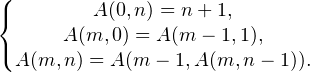Find the recursion depth.

Block-scheme of the solution:

C++ implementation:

#include<iostream>
using namespace std;
int depth;

int Ackerman(int m, int n)//Ackerman function
{
depth++;//calculate the recursion depth
if (m == 0) return n + 1;//end of the recursion
else
{
if (n == 0) return Ackerman(m - 1, 1);//recursy
else return Ackerman(m - 1, Ackerman(m, n - 1));
}
}
void main()
{
int m, n, result;
cout << "Input m > 0, n > 0:" << endl;
cin >> m >> n;//input m & n

if (n >= 0 && m >= 0)//n & m must be >= 0
{
result = Ackerman(m, n);//calculate solution, call Accerman function
cout << "Ackerman(" << m << "," << n << ")=" << result << endl << "recursion depth = " << depth << endl;//output m, n, solution, recursion depth
}
else cout << "m or n < 0" << endl;//if m & n < 0
system("pause");//pause console
}



Screenshots: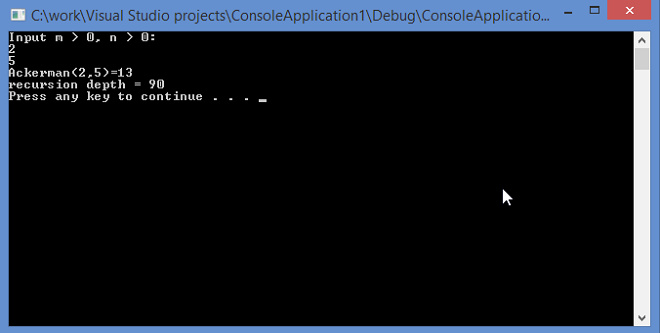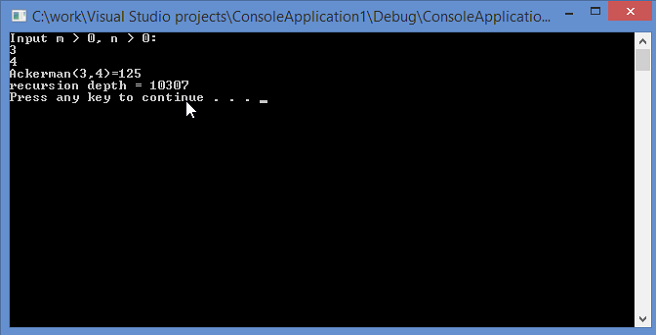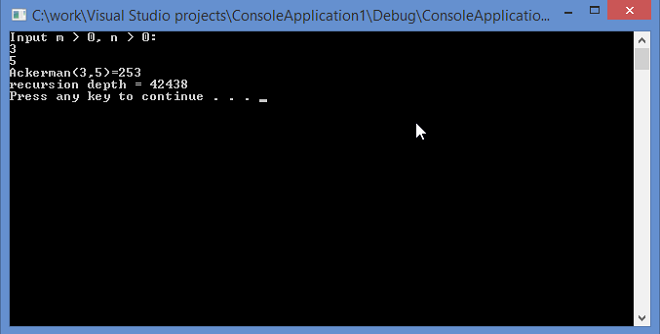Let’s use the table of values of Ackerman function from the Wikipedia to ensure that the program works properly: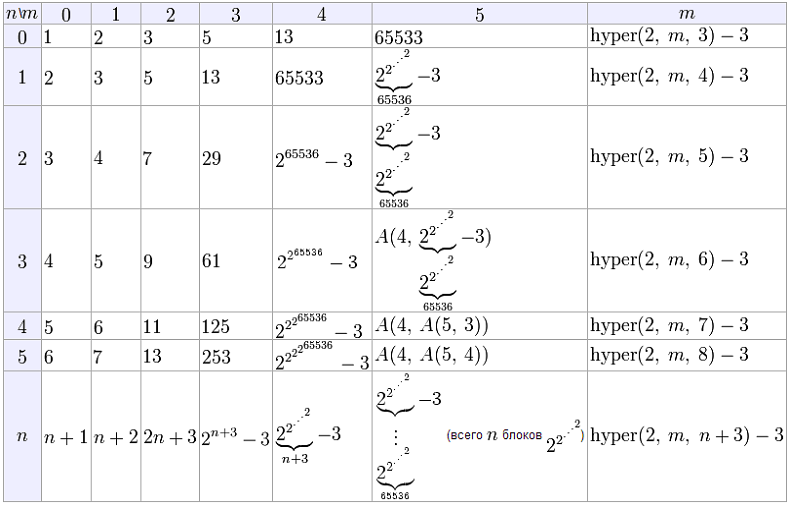This sample is not the single. You have the ability to get acquainted with more sample assignments that concern various disciplines on AssignmentShark. Our homework help service was founded to be helpful for students. Therefore, we try to post useful materials that can help students with their studying. You can look through our articles with tips and recommendations and our sample assignments any time you are in need. If it is not enough, you can also apply for assignment help to our experts. They will help you to complete your assignments quickly and correctly. Each of them is an experienced expert in the specific field. Our service is absolutely safe. There’s no need to worry about your confidentiality. Try our service right now and get your web assignment sample completed by one of our experts! We are available 24/7!

Help me with my assignment. – With ease!